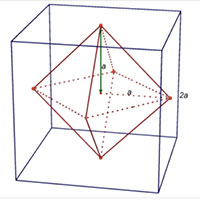# u3a - Maths Challenge - Week 3

## Maths Challenge - Week 3

• Published : 24 April 2020
• Views: 2906

Welcome to week 3 of our weekly maths challenge, with problems and puzzles posed by Gordon Burgin, Andrew Holt and the U3A Maths and Stats Subject Adviser - David Martin.  Solutions to Week 2 are at the bottom of the page

Check back each week for the solutions and let us know how you get on by contacting National Office. New maths puzzles will go up each week.

## Week 3

Question 1.
In a batch of 8 correctly manufactured balls , a faulty, slightly lighter ball has
weights how could the lighter ball be identified and what is the minimum
number of weighings needed?

Question 2.
In ‘Die Hard with a Vengeance’ Bruce Willis and Samuel L. Jackson were called
upon to measure out exactly 4 gallons of water at a fountain to defuse a bomb.
They were to use just a 3 gallon container and a 5 gallon container. How would
you have solved this puzzle? Can you find a way of measuring out all amounts
from 1 gallon to 8 gallons?

Question 3.
Show that if p is a prime greater than 3 then p2 – 1 is divisible by 24

Question 4.
What is the probability of getting a one pair hand in poker?

## Week Two - Solutions

Question 1.
If the cold tap alone would fill a bath in 20 minutes and the hot tap alone would fill a bath in 30 minutes, how many minutes would it take to fill the bath with both the cold and hot taps on?
Solution
The cold tap fills at the rate of 3 baths per hour while the hot tap fills at the rate of 2 baths per hour. The cold and hot taps together would fill at the rate of 3+2 = 5 baths per hour, hence the time to fill one bath with both taps on would be one fifth of an hour, that is 12 minutes.

Question 2.
The six angles of two different triangles are listed in decreasing order. The list starts with 115, 85, 75 and 35. What is the last angle in the list?
Solution
115 + 85 = 200; 115 + 75 = 190; so 85and 75 cannot be in a triangle with 115.
The remaining angle in the triangle with 85 and 75 is 20.
20 is less than (180 – 115 – 35) = 30, so 20° is the last angle in the list.

Question 3.
Eight pens cost £10 and some pence while eleven more cost £26 and some pence. What is the cost of a pen?
Solution
Let the cost of a pen be p pence.
8 x 125 = 1000, 8 x 138 = 1104; so p is at least 126 and no more than 137
19 x 137 = 2603; so p is at least 137
Hence p = 137 and cost of a pen is £1.37.

Question 4.

An octahedron is drawn by connecting the centres of the faces of a cube. What is the ratio of the volume of the octahedron to that of the cube?
SolutionSuppose the cube side length equals 2a.
The octahedron is made up of an upper pyramid and a lower pyramid. The height h of each pyramid equals a. By Pythagoras the base of the pyramids is a square of side length √2a. So, the surface area B of the base equals 2a2.
Volume of octahedron
= volume of upper pyramid + volume of lower pyramid
= 2Bh/3
= 4a3/3
Volume of cube = 8a3
Ratio of volume of octahedron to volume of cube = (4a3/3)/8a3 = 1/6.